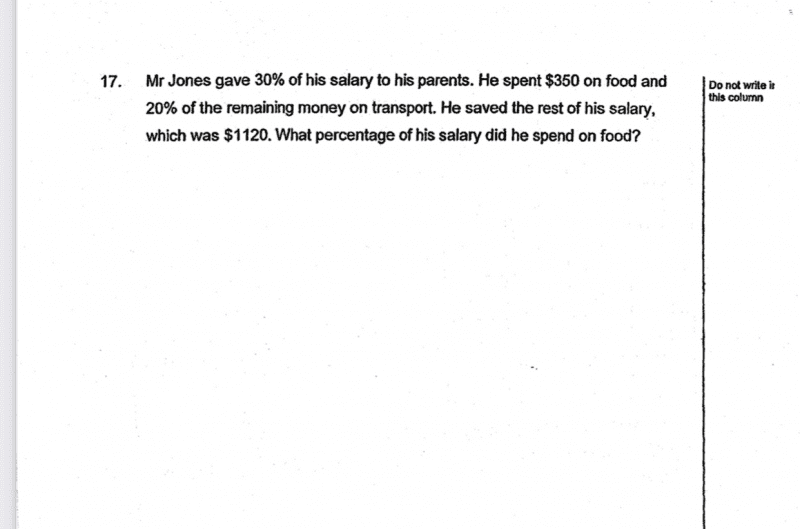QuestionLet x be the amount of salary spent on transport and saved.

80% x = \$1120, so x = \$1400

Including food, he would have \$1400+\$350 = \$1750

This is 70% of his salary.

So his salary is 1750 * 100 / 70 = 2500

Therefore % of salary spent on food = 350/2500 * 100 = 14% #